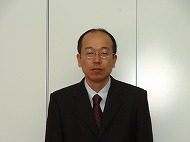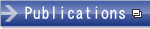HOME > Faculty and Staff > Faculty

## Faculty and Staff

﻿

### Yoshihito KohsakaDegree
Ph. D of Science (Hokkaido University, 2000)
Educational Contents
Undergraduate Course: Calculus A/B, Linear Algebra A/B, Applied Mathematics 4
Research Interests
My research interests are the mathematical analysis of the nonlinear partial differential equations and the free boundary problems that appear in the material science and the biophysics. In particular, I am interested in the geometric evolution equations (for example, mean curvature flow, surface diffusion equation, Helfrich flow) that describe the motion of interfaces. I try to analyze the motion of interfaces mathematically by investigating the properties of solutions of these geometric evolution equations.
Message for Students
When we study a phenomenon that is observed in separate fields, we sometimes find that it can be represented by the same mathematical principle. Mathematics is a basis of the analysis of various phenomena and relates the different phenomena in principle. I hope that you study such aspects of mathematics.
Research Areas
Nonlinear analysis, Partial differential equations
Affiliations
The Mathematical Society of JapanPageTop